# Arduino串口数据可视化方法

laokugonggao

0收藏

Arduino IDE: 1.8.5
Arduino开发板：UNO

Arduino可以方便地操作传感器获得传感器数据，获取数据之后我们比较关心的是数据可视化Arduino自带有串口监视器和串口绘图器，我还找到了其他3种适合于arduino串口数据绘图的工具，这篇博客也当做个记录，分别介绍这4种绘图方法，传感器数据是上一篇博客介绍的K型热电偶采集的温度。

（1）IDE自带的串口绘图器

``````#include <MAX6675_Thermocouple.h>

#define SCK_PIN 3 // 模块上的SCK口连接到 pin3
#define CS_PIN  4 // 模块上的CS口连接到 pin4
#define SO_PIN  5 // 模块上的SO口连接到 pin5
// 不要忘了模块上的5v和GND要连接到arduino开发板

MAX6675_Thermocouple* thermocouple = NULL;

// the setup function runs once when you press reset or power the board
void setup() {
Serial.begin(9600); //设置串口波特率9600
thermocouple = new MAX6675_Thermocouple(SCK_PIN, CS_PIN, SO_PIN);
}

// the loop function runs over and over again forever
void loop() {
double celsius = thermocouple->readCelsius(); // 摄氏度

Serial.println(celsius);  // println打印会自动添加'\r\n'，可以在串口绘图器显示
delay(500);// 延时500ms
}
``````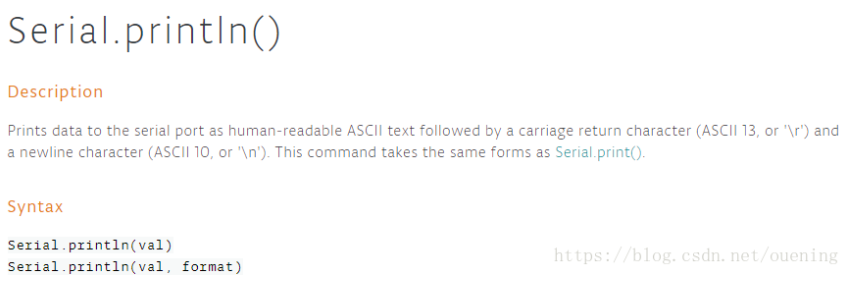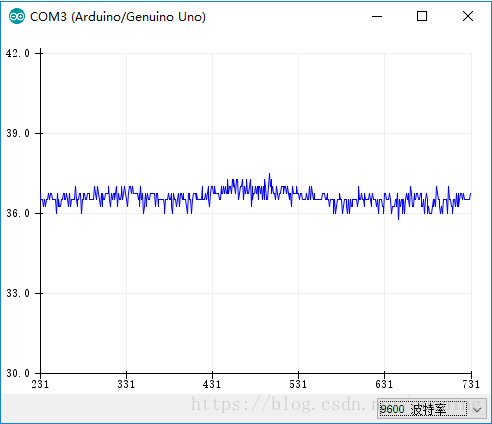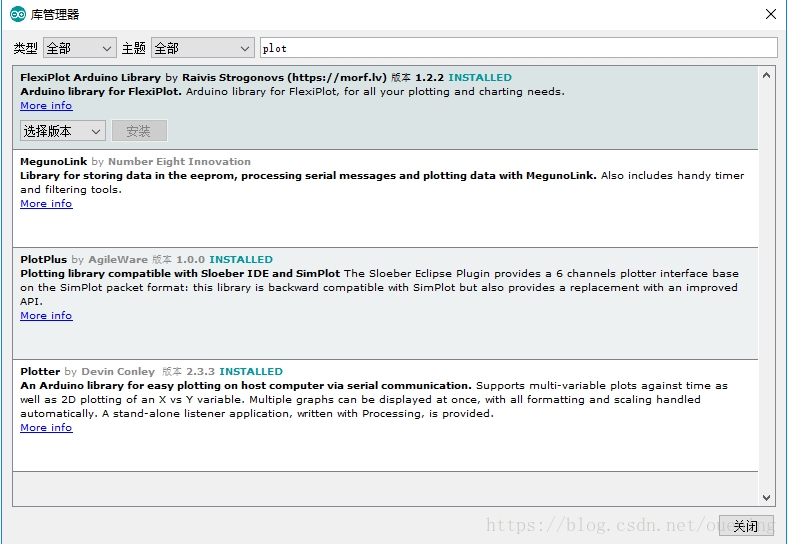（2）Plotter

``````#include <MAX6675_Thermocouple.h>
#include "Plotter.h"
double x;
Plotter p;

#define SCK_PIN 3 // 模块上的SCK口连接到 pin3
#define CS_PIN  4 // 模块上的CS口连接到 pin4
#define SO_PIN  5 // 模块上的SO口连接到 pin5
// 不要忘了模块上的5v和GND要连接到arduino开发板

MAX6675_Thermocouple* thermocouple = NULL;

// the setup function runs once when you press reset or power the board
void setup() {
p.Begin();  // 创建一个plotter
p.AddTimeGraph( "Temperature of K-type thermalcouple", 100, "temperature", x ); // 添加时间图表
// 不需要设置波特率
thermocouple = new MAX6675_Thermocouple(SCK_PIN, CS_PIN, SO_PIN);
}

// the loop function runs over and over again forever
void loop() {
double celsius = thermocouple->readCelsius(); // 摄氏度
x = celsius;
p.Plot();
//  Serial.println(celsius);  // println打印会自动添加'\r\n'，可以在串口绘图器显示
delay(500);// 延时500ms
}
``````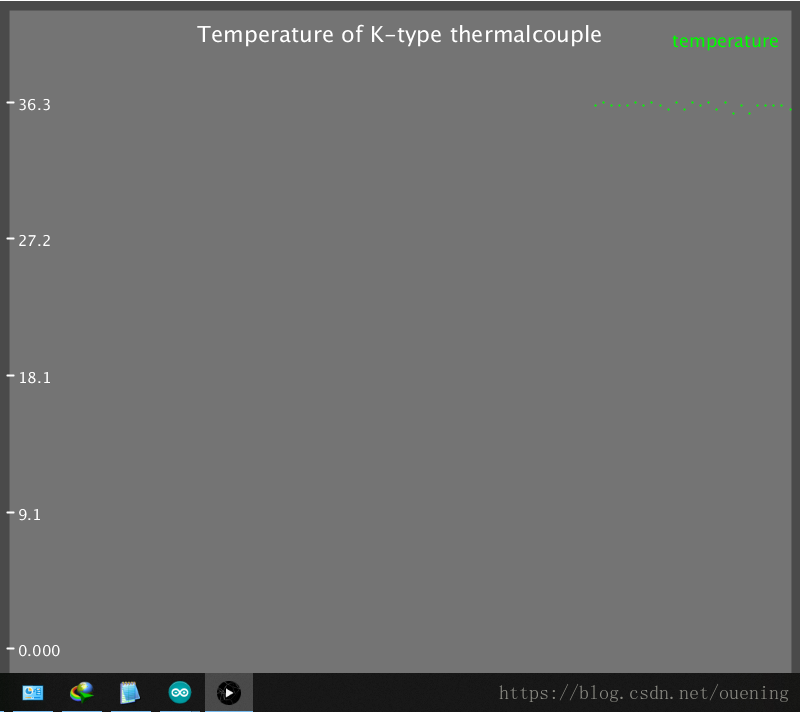（3）FlexiPlot

（3）PlotPlus，兼容SimPlot

``````#include <MAX6675_Thermocouple.h>
#include <PlotPlus.h>

#define SCK_PIN 3 // 模块上的SCK口连接到 pin3
#define CS_PIN  4 // 模块上的CS口连接到 pin4
#define SO_PIN  5 // 模块上的SO口连接到 pin5
// 不要忘了模块上的5v和GND要连接到arduino开发板

MAX6675_Thermocouple* thermocouple = NULL;

// the setup function runs once when you press reset or power the board
void setup() {
Serial.begin(9600); //设置串口波特率9600
thermocouple = new MAX6675_Thermocouple(SCK_PIN, CS_PIN, SO_PIN);
}

// the loop function runs over and over again forever
void loop() {
double celsius = thermocouple->readCelsius(); // 摄氏度
plot(celsius);
//  Serial.println(celsius);  // println打印会自动添加'\r\n'，可以在串口绘图器显示
delay(500);// 延时500ms
}
``````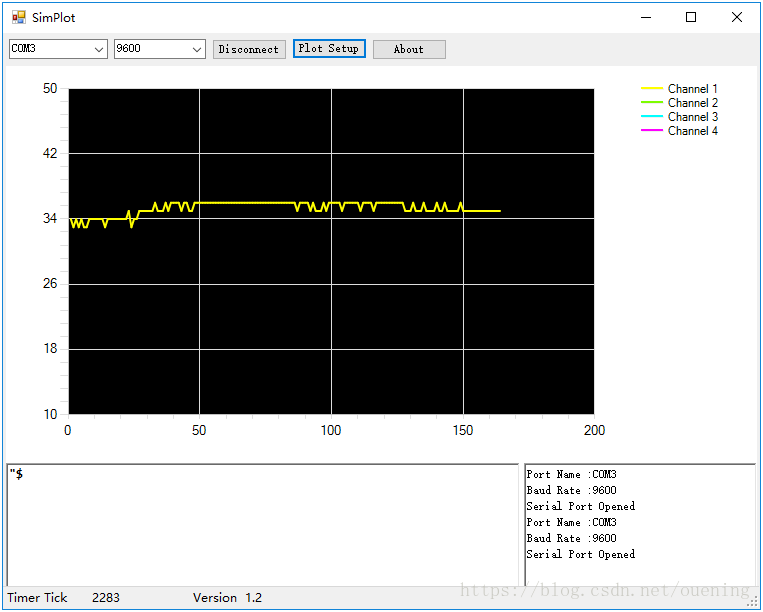帖子
视频
声望
粉丝
相关问题
社区精华内容